Download Presentation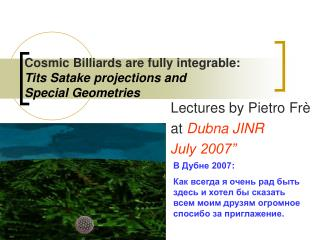Cosmic Billiards are fully integrable: Tits Satake projections and Special Geometries

# Cosmic Billiards are fully integrable: Tits Satake projections and Special Geometries - PowerPoint PPT Presentation

Cosmic Billiards are fully integrable: Tits Satake projections and Special Geometries Lectures by Pietro Frè at Dubna JINR July 2007” В Дубне 2007 : Как всегда я очень рад быть здесь и хотел бы сказать всем моим друзям огромное спосибо за приглажение . Introduction to cosmic billiardsI am the owner, or an agent authorized to act on behalf of the owner, of the copyrighted work described.
Download Presentation## Cosmic Billiards are fully integrable: Tits Satake projections and Special Geometries

An Image/Link below is provided (as is) to download presentation

Download Policy: Content on the Website is provided to you AS IS for your information and personal use and may not be sold / licensed / shared on other websites without getting consent from its author.While downloading, if for some reason you are not able to download a presentation, the publisher may have deleted the file from their server.

- - - - - - - - - - - - - - - - - - - - - - - - - - E N D - - - - - - - - - - - - - - - - - - - - - - - - - -
Presentation Transcript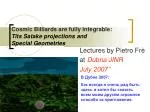### Cosmic Billiards are fully integrable:Tits Satake projections and Special Geometries

Lectures by Pietro Frè

at Dubna JINR

July 2007”

В Дубне 2007:

Как всегда я очень рад быть здесь и хотел бы сказать всем моим друзям огромное спосибо за приглажение.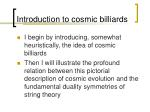Introduction to cosmic billiards
• I begin by introducing, somewhat heuristically, the idea of cosmic billiards
• Then I will illustrate the profound relation between this pictorial description of cosmic evolution and the fundamental duality symmetries of string theory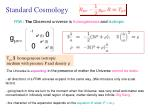Standard Cosmology

g=

-1

0

a2 (t)

a2 (t)

0

a2 (t)

FRW : TheObserved universe is homogeneous and isotropic

Tmn \$ homogeneous isotropic

medium with pressure P and density r

• The Universe is expanding, in the presence of matter the Universe cannot be static,
• all directions of a FRW universe expand in the same way, (We introduce only one scale factor)
• going back in time we turn to the moment when the Universe was very small and matter was concentrated in infinetely small region of space, matter density was infinite: Big Bang,
• the character of the expansion depends on the equation of state: P = w 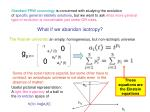g=

0

-1

a12 (t)

a22 (t)

0

a32 (t)

h2

Useful pictorial representation:

A light-like trajectory of a ball in

the lorentzian space of

h3

hi(t)= log[ai(t)]

h1

Standard FRW cosmology is concerned with studying the evolution

of specific general relativity solutions, but we want to ask what more general

type of evolution is conceivable just under GR rules.

What if we abandon isotropy?

The Kasner universe: an empty, homogeneous, but non-isotropic universe

Some of the scale factors expand, but some other have to contract: an anisotropic universe is not static even in the absence of matter!

These equations are the Einstein equations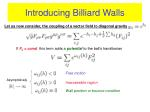Let us now consider, the coupling of a vector field to diagonal gravity

Introducing Billiard Walls

If Fij = const this term adds a potential to the ball’s hamiltonian

Free motion

Asymptoticaly

Inaccessible region

Wall position or bounce condition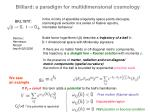cosmological evolution is a series of Kasner epochs,

mixmaster behaviour

BKL1970’:

Scale factor logarithms hi(t) describe a trajectory of a ball in

D-1 dimensional space with Minkovsky signature.

Damour,

Henneaux,

Nicolai

hep-th/0212256

If there are no matter fields or off-diagonal metric components,

this trajectory is a straight line – Kasner solution with momenta pi

In the presence of matter, radiation and non-diagonal

metric components (spatial curvature)

the motion of the ball is bounded by exponential potential walls

We saw

an example

If Fij = const this term adds a potential to the ball’s hamiltonian

Free motion (Kasner epoch)

Inaccessible region

Cij2>0 ! the potential is repulsive!

Wall position or bounce condition

Billiard: a paradigm for multidimensional cosmology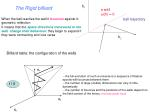h1

a wall

ω(h) = 0

h2

H1

t ! 0

H3

H2

The Rigid billiard

When the ball reaches the wall it bounces against it:

geometric reflection

ball trajectory

It means that the space directions transverse to the wallchange their behaviour: they begin to expand if they were contracting and vice versa

Billiard table: the configuration of the walls

-- the full evolution of such a universe is a sequence of Kasner epochs with bounces between them

-- the number of large (visible) dimensions can vary in time dynamically

-- the number of bounces and the positions of the walls depend on the field content of the theory: microscopical input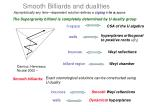Damour, Henneaux,

Nicolai 2002 --

Smooth Billiards and dualities

Asymptotically any time—dependent solution defines a zigzag in ln ai space

The Supergravity billiard is completely determined by U-duality group

h-space

CSA of the U algebra

hyperplanes orthogonal

to positive roots(hi)

walls

bounces

Weyl reflections

billiard region

Weyl chamber

Exact cosmological solutions can be constructed using

U-duality

Smooth billiards:

bounces

Smooth Weyl reflections

walls

Dynamical hyperplanes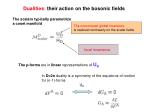The noncompact global invariance

is realized nonlinearly on the scalar fields

local invariance

are in linear representations of UD

The p-forms

Dualities: their action on the bosonic fields

The scalars typically parametrize

a coset manifold

In D=2n duality is a symmetry of the equations of motion

for (n-1)-forms

UDi gMN = 0

SL(N)/SO(N)

Supergravities in different dimensions are connected by

dimensional reduction

a , gMN , … M = 0, .., D=1

a ( g , g i , gi,j )

= 0,.. , d

i = i,..,N

More scalars and more global symmetries !### Smooth supergravity and superstring billiards....

THE MAIN IDEA

from a D=3 viewpoint

P. F. ,Trigiante, Rulik, Gargiulo, Sorin

Van Proeyen and Roossel

2003,2004, 2005

various papersStarting from D=3(D=2 and D=1, also)all the (bosonic) degrees of freedom are scalars

The bosonic Lagrangian of any Supergravity, can be reduced in D=3, to a gravity coupled sigma modelU

D>3 SUGRA

D>3 SUGRA

dimensional oxidation

UmapsD>3 backgrounds

into D>3 backgrounds

dimensional

reduction

Not unique: classified by different embeddings

U

D=3 sigma model

D=3 sigma model

Field eq.s reduce to Geodesic equations on

Solutions are classified by abstract subalgebras

Since all fields are chosen to depend only on one coordinate, t = time, then we can just reduce everything to D=3, D=2 or D=1. In these dimensions every degree of freedom (bosonic) is a scalar

Time dep. backgrounds

NOMIZU OPERATOR

SOLVABLE ALGEBRA

Nomizu connection = LAX PAIR

Representation. INTEGRATION!With this machinery.....
• We can obtain exact solutions for time dependent backgrounds
• We can see the bouncing phenomena (=billiard)

We have to extend the idea to lower supersymmetry # QSUSY < 32 and...

• We do not have to stop at D=3. For time dependent backgrounds we can start from D=2 or D=1
• In D=2 and D=1 we have affine and hyperbolic Kac Moody algebras, respectively.....!How to build the solvable algebra

Given the Real form of the algebra U, for each positive root

there is an appropriate step operator belonging to such a real formMaximal Susy implies Er+1 series

Scalar fields are associated with positive roots or Cartan generatorsFrom the algebraic view point.....
• Maximal SUSY corresponds to...
• MAXIMALLY non-compact real forms:
• i.e. SPLIT ALGEBRAS.
• This means:
• All Cartan generators are non compact
• Step operators E2 Solv , 82+
• The representation is completely real
• The billiard table is the Cartan subalgebra of the isometry group!### Let us briefly survey

The use of the solvable parametrization as a machinary to obtain solutions,

in the split caseThe general integration formula
• Initial data at t=0 are
• A) , namely an element of the Cartan subalgebra determining the eigenvalues of the LAX operator
• B) , namely an element of the maximal compact subgroup

Then the solution algorithm generates a uniquely defined time dependent

LAX operatorProperties of the solution
• For each element of the Weyl group
• The limits of the LAX operator at t=§1 are diagonal
• At any instant of time the eigenvalues of the LAX operator are constant1, ...,n
• where wiare the weights of the representation to which the Lax operator is assigned.Disconnected classes of solutions

Property (2) and property (3) combined together imply that the two asymptotic values L§1 of the Lax operator are necessarily related to each other by some element of the Weyl group

which represents a sort of topological charge of the solution:

The solution algorithm induces a map:A plotted example with SL(4,R)/O(4)
• The U Lie algebra is A3
• The rank is r = 3.
• The Weyl group is S4 with 4! elements
• The compact subgroup H = SO(4)

The integration formula can be easily encoded into a computer programme and for any choice of the eigenvalues

and for any choice of the group element2 O(4)

The programme CONSTRUCTS the solution2+3

1+2 +3

3

2

1+2

1

Plots of the (integrated) Cartan Fields along

the simple roots

This solution has four bounces### Let us now introduce more structure of SUGRA/STRINGTHEORY

The first point:

Less SUSY (NQ < 32)

and

non split algebrasWHAT are these new manifolds (split!) associated with the known non split ones....???The Billiard Relies on Tits Satake Theory
• To each non maximally non-compact real form U (non split) of a Lie algebra of rank r1 is associated a unique subalgebra UTS½ U which is maximally split.
• UTS has rank r2 < r1
• The Cartan subalgebra CTS½ UTS is the true billiard table
• Walls in CTS now appear painted as a memory of the parent algebra USeveral roots of the higher system have the same projection.

These are painted copies of the same wall.

The Billiard dynamics occurs in the rank r2 system

Projection

root system

of rank r1

rank r2 < r1

root latticer – split rank

compact roots

non compact roots

root pairs

Compact

simple roots

define a

sugalgebra

Hpaint

To say it in a more detailed way:

Non split algebras arise as duality algebras in non maximal supergravities N< 8

Under the involutive automorphism  that defines the non split real section

Non split real algebras are represented by Satake diagrams

For example, for N=6 SUGRA

we have E7(-5)Why is it exciting?
• Since the Nomizu connection depends only on the structure constants of the Solvable Lie algebraD=4

D=3

Paint group in diverse dimensions

The paint group survives under dimensional reduction, that adds only

non-compact directions to the scalar manifold

It means that the Tits Satake projection commutes with the dimensional reduction### More about the Tits Satake projection

It is a projection....

Supergravity Models fall into Universality classesClassification

of special geometries,

namely of the

scalar sector of supergravity with

8 supercharges

In D=5,

D=4

and D=3

D=5

D=4

D=3The paint group

The subalgebra of external automorphisms:

is compact and it is the Lie algebra of the paint group### And now let us go the next main point..

Kac Moody ExtensionsAffine and Hyperbolic algebrasand the cosmic billiard

(Julia, Henneaux, Nicolai, Damour)

• We do not have to stop to D=3 if we are just interested in time dependent backgrounds
• We can step down to D=2 and also D=1
• In D=2 the duality algebra becomes an affine Kac-Moody algebra
• In D=1 the duality algebra becomes an hyperbolic Kac Moody algebra
• Affine and hyperbolic symmetries are intrinsic to Einstein gravityUniversal,

comes

from Gravity

Comes from vectors in D=4

Symplectic metric in d=2

Symplectic metric in 2n dim

Structure of the Duality Algebra in D=3(P.F. Trigiante, Rulik and Gargiulo 2005)Let me remind you…
• about the relation between
• the Cartan presentation
• and the Chevalley Serre presentation of a Lie algebraCartan subalgebra (CSA)

Root generators

Simple roots i

Cij

Dynkin diagram

Cartan matrix

Chevalley-Serre presentation

Cartan presentationUD=4

W

TA

(L0 L+ L-)

(W+ W-)

new symmetries

The new Chevalley-Serre triplet: we take the highest weight of the

symplectic representation hThe Kac Moody extension of the D=3 Duality algebra

In D=2 the duality algebra becomes the Kac Moody extension of the algebra in D=3.

Why is that so?The reason is...
• That there are two ways of stepping down from D=4 to D=2
• The Ehlers reduction
• The Matzner&Misner reduction
• The two routes give two different lagrangians with two different finite algebra of symmetries
• There are non local relations between the fields of the two lagrangians
• The symmetries of one Lagrangian have a non local realization on the other and vice versa
• Together the two finite symmetry algebras provide a set of Chevalley generators for the Kac Moody algebra### Let us review

The algebraic mechanism of this extensionDuality algebrasfor diverse N(Q) from D=4 to D=3

N=8

E7(7)

E8(8)

SO*(12)

E7(-5)

N=6

N=5

SU(1,5)

E6(-14)

N=4

SL(2,R)£SO(6,n)

SO(8,n+2)

N=3

SU(3,n) £ U(1)Z

SU(4,n+1)3

4

5

6

7

What happens for D<3?

Exceptional E11- D series for N=8 give a hint

0

2

9

D

8

SL(2)

+ SL(3)

GL(2,R)

SO(5,5)

SL(5)

Julia 1981

UD

E8

E3

E9 = E8Æ

E7

E6

E5

E4UD=4

W

0

UD=4

W1

W2

0

This extensions is affine!

The new affine triplet: (LMM0, LMM+, LMM-)

The new triplet is connected to the vector root with a single line,

since the SL(2)MM commutes with UD=4

2 exceptions: pure D=4 gravity and N=3 SUGRA

0

1D=4

D=3

D=2

N=8

E7(7)

E8(8)

E9(9)

SO*(12)

E7(-5)

N=6

E7

N=5

SU(1,5)

E6(-14)

E6

N=4

SL(2,R)£SO(6,n)

SO(8,n+2)

SO(8,n+2)

N=3

SU(3,n) £ U(1)Z

SU(4,n+1)Conclusions
• The algebraic structure of U duality algebras governs many aspects of String Theory
• In particular it is responsible for the cosmic billiard paradigma of multidimensional cosmologies
• The integrability of the maximal split case has to be extended to the non maximal split cases taking advantage of TS projection (work in progress)
• The exact integrability in D=3 has to be extended to the affine and hyperbolic cases
• This is for ungauged supergravities……Gauged Supergravities
• Gauging is equivalent to introducing fluxes
• In gauged supergravities we have -models with potentials…
• The integrability of these cases is an entirely new chapter….
• May be for next year seminar….!